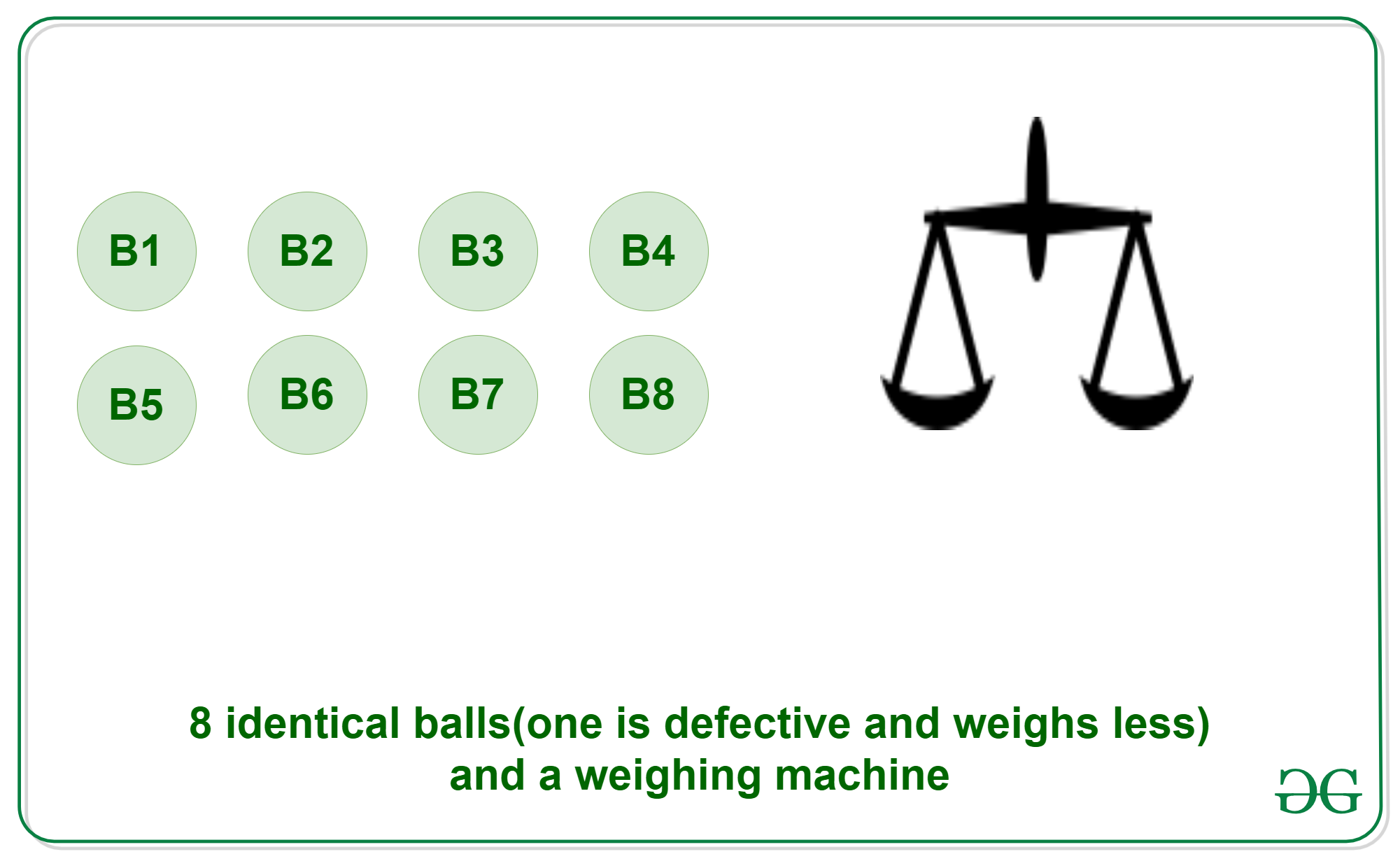Puzzle | 8 balls problem

• Difficulty Level : Easy
• Last Updated : 03 Dec, 2019

Puzzle: You are provided with 8 identical balls and a measuring instrument. 7 of the eight balls are equal in weight and one of the eight given balls is defective and weighs less. The task is to find the defective ball in exactly two measurements.Solution:

Now let’s analyse the three conditions to find the defective ball: